• 数字信号载波传输OFDM0 OFDM基本原理1 OFDM调制2 OFDM解调3 OFDM仿真 0 OFDM基本原理 OFDM（Orthogonal Frequency Division Multiplexing）即正交频分复用技术，实际上OFDM是MCM（Multi Carrier Modulation），...

0 OFDM基本原理

OFDM（Orthogonal Frequency Division Multiplexing）即正交频分复用技术，实际上OFDM是MCM（Multi Carrier Modulation）多载波调制的一种。通过频分复用实现高速串行数据的并行传输，它具有较好的抗多径衰弱的能力，能够支持多用户接入。在LTE、5G NR、802.11ax通信协议的物理层中都用到了OFDM技术。

OFDM主要思想是：将信道分成若干正交子信道，将高速数据信号转换成并行的低速子数据流，调制到在每个子信道上进行传输。正交信号可以通过在接收端采用相关技术来分开，这样可以减少子信道之间的相互干扰(ISI)。每个子信道上的信号带宽小于信道的相关带宽，因此每个子信道上可以看成平坦性衰落，从而可以消除码间串扰，而且由于每个子信道的带宽仅仅是原信道带宽的一小部分，信道均衡变得相对容易。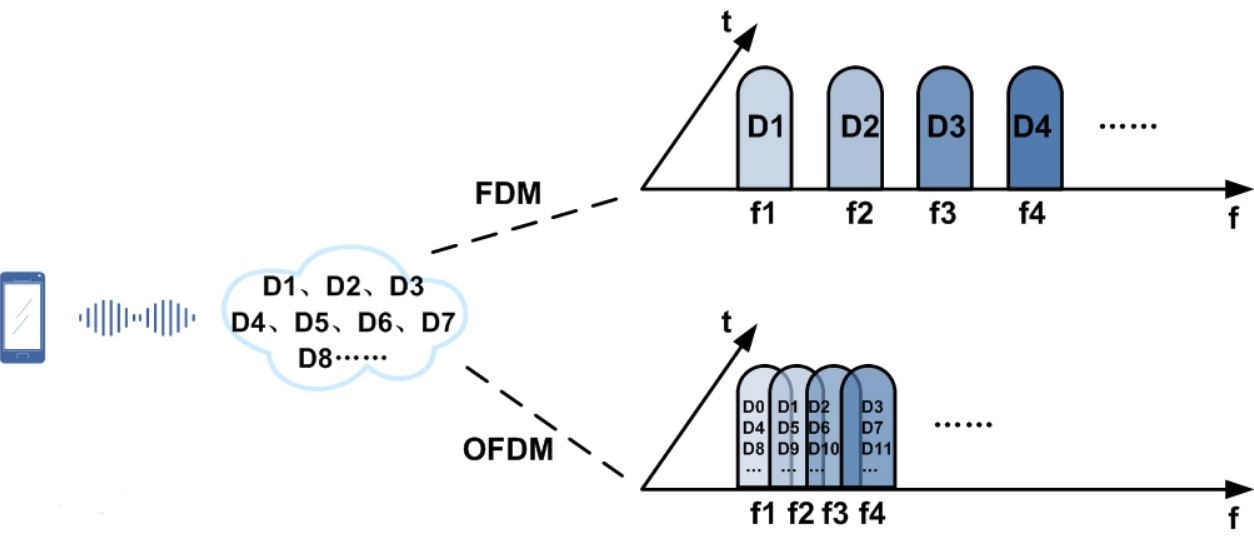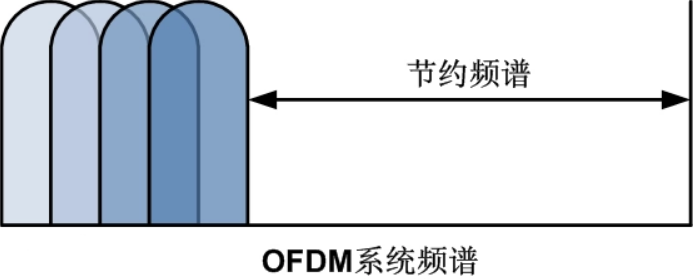OFDM中的各个载波是相互正交的，每个载波在一个符号时间内有整数个载波周期，每个载波的频谱最大值点和相邻载波的零点重叠，这样便减小了载波间的干扰。由于载波间有部分重叠，所以它比传统的FDMA提高了频带利用率。

OFDM符号：如果系统带宽为20M，那么系统包含100个RB,每个RB包含12个子载波，即一个OFDM符号上共有1200个子载波。同时，一个OFDM符号包含的比特数也与资源元素选用的调制方式有关系，如果所有资源单元（子载波）都选用64QAM调制，则一个资源元素包含6个bit。这样，一个OFDM符号的比特数=100126 bit=7200 bit。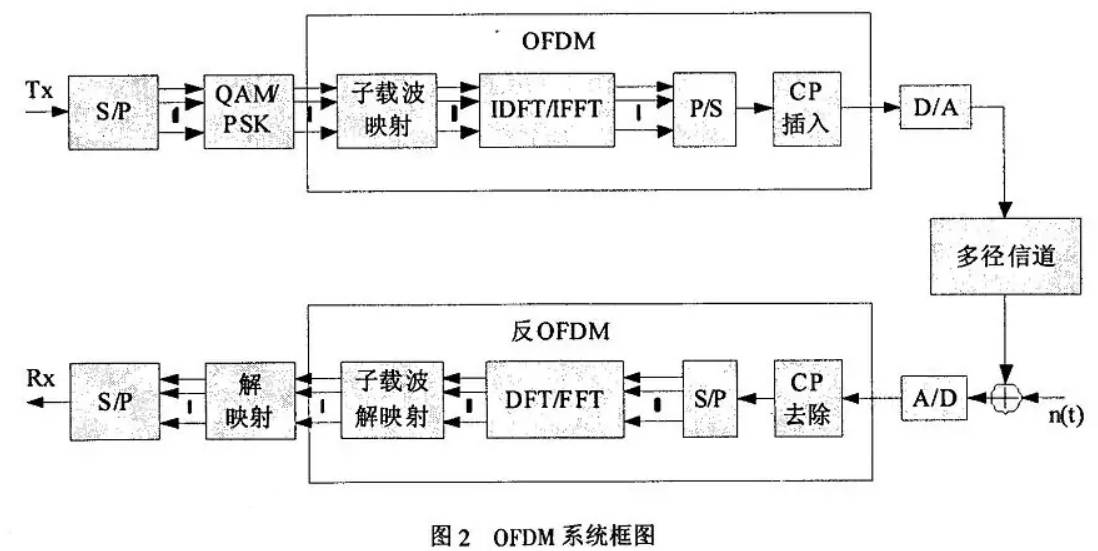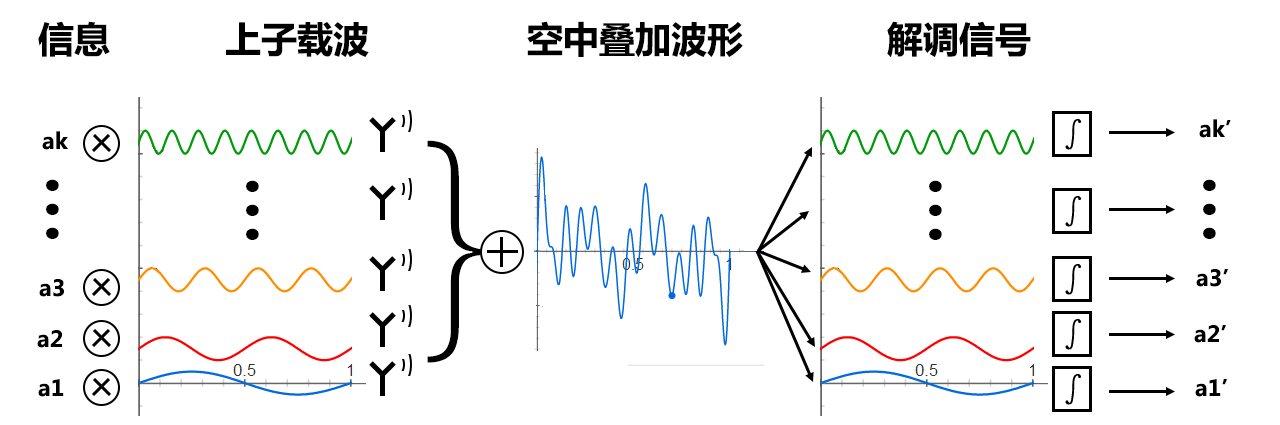IDFT、IFFT

DFT、FFT

3 OFDM仿真

MATLAB程序

clc;
clear;
close all;

M  = 8;			% 子载波数
fc   = 1e6;		% 主载波频率/Hz
fsub = 1e3;		% 子载波频率间隔
fsig = fc:fsub:fc+(M-1)*fsub; % 频率序列

T  = 0.001;		% 子载波持续时间
fs = 10e6;		% 采样频率/Hz
ts = 1/fs;		% 采样时间间隔
t  = 0:ts:T-ts;	% 一个符号周期的时间矢量

% 载波信号
c  = zeros(M,length(t));
NN = length(t)*16;
XN = zeros(M,NN);
f0 = fs/NN;
f  = (0:NN-1)*f0;
for k = 1:M
c(k,:)  = exp(1j*2*pi*fsig(k)*t);
XN(k,:) = fft(c(k,:),NN);
end

figure;
plot(f,abs(XN(1,:)), f,abs(XN(2,:)), f,abs(XN(3,:)), f,abs(XN(4,:)), f,abs(XN(5,:)), f,abs(XN(6,:)), f,abs(XN(7,:)), f,abs(XN(8,:)));
legend('1000kHz子载波', '1001kHz子载波', '1002kHz子载波', '1003kHz子载波', '1004kHz子载波', '1005kHz子载波', '1006kHz子载波', '1007kHz子载波');
axis([995e3 1012e3 -inf inf]);
title('频域中 子载波分布图');
ylabel('幅度');
xlabel('频率/Hz');

symbol = M;
msg  = randi([0 1],1,symbol);% 并行发送8bit数据
% msg  = [1 1 0 1 1 1 0 1];% 并行发送8bit数据
tx = zeros(1,length(t));
for k = 1:length(msg)
tx = tx + msg(k)*c(k,:);% 子载波叠加
end
XN_tx = fft(tx,NN);
disp(['发送数据： ' num2str(msg)]);

% 空中信道传输
sigma = sum(abs(tx))/length(tx) * 0.9;
rx    = tx + sigma*rand(1,length(tx));% 加入AWGN（实际上只影响直流分量）
XN_rx = fft(rx,NN);

% OFDM解调
msg_demodulation = zeros(1,symbol);
for k = 1:symbol
if(abs(XN_rx(16001 + 16*(k-1))) > 5e3) % 使用FFT结果来解调
msg_demodulation(1,k) = 1;
end
end
disp(['收到数据： ' num2str(msg_demodulation)]);

bit_error_cnt = 0;
for k = 1:symbol
if(msg_demodulation(k) ~= msg(k))
% 当判定的接收比特与发送比特不一致时，认为判定错误
bit_error_cnt = bit_error_cnt + 1;
end
end
bit_error_percent = bit_error_cnt/symbol;
disp(['误码率： ' num2str(bit_error_percent)]);

figure;
subplot(2,1,1);plot(t,real(tx));axis([-inf inf -inf inf]);title('OFDM发送信号 时域图');
ylabel('幅度');
xlabel('时间/s');
subplot(2,1,2);plot(t,real(rx));axis([-inf inf -inf inf]);title('OFDM接收信号 时域图');
ylabel('幅度');
xlabel('时间/s');

figure;
subplot(2,1,1);plot(f,abs(XN_tx));axis([995e3 1012e3 -inf inf]);title('OFDM发送信号 频谱');
ylabel('幅度');
xlabel('频率/Hz');
subplot(2,1,2);plot(f,abs(XN_rx));axis([995e3 1012e3 -inf inf]);title('OFDM接收信号 频谱');
ylabel('幅度');
xlabel('频率/Hz');

结果：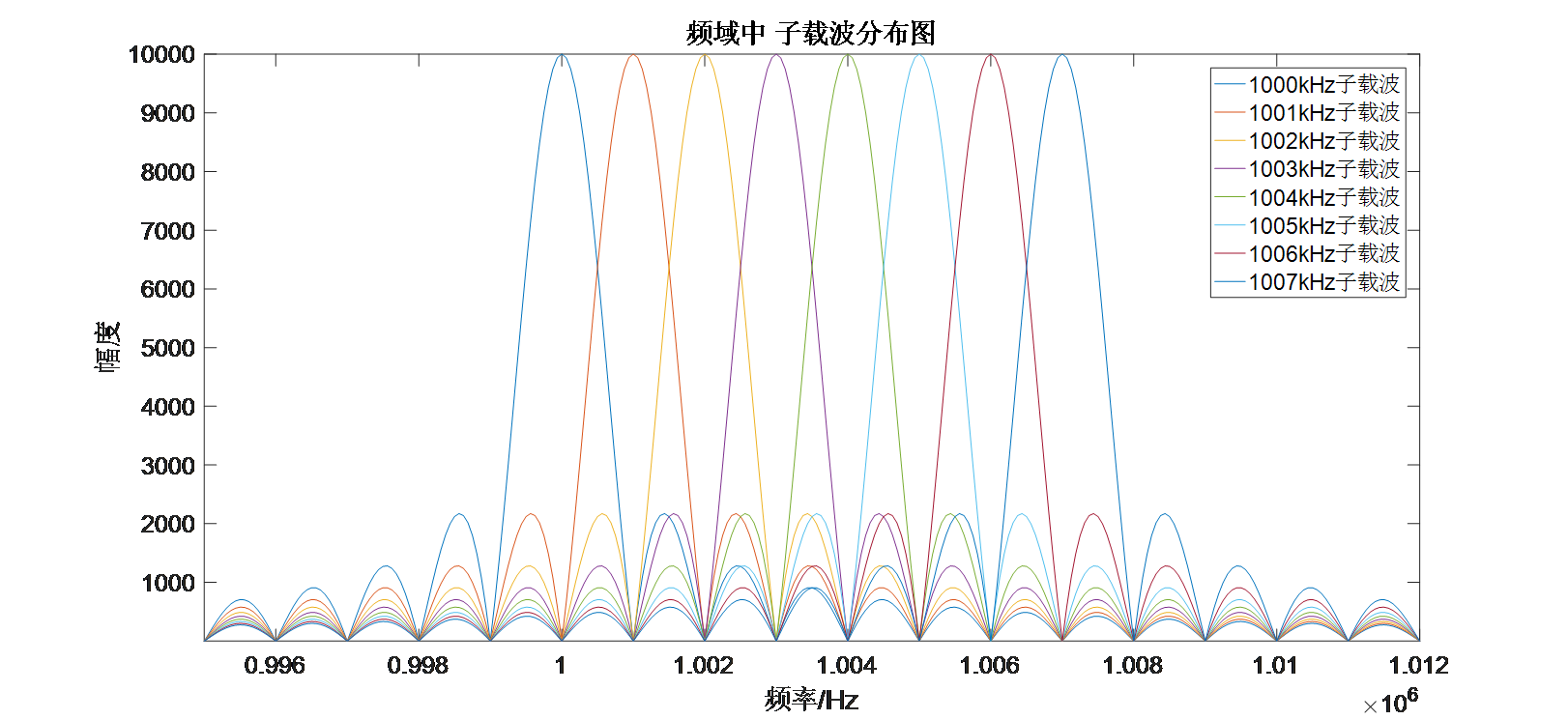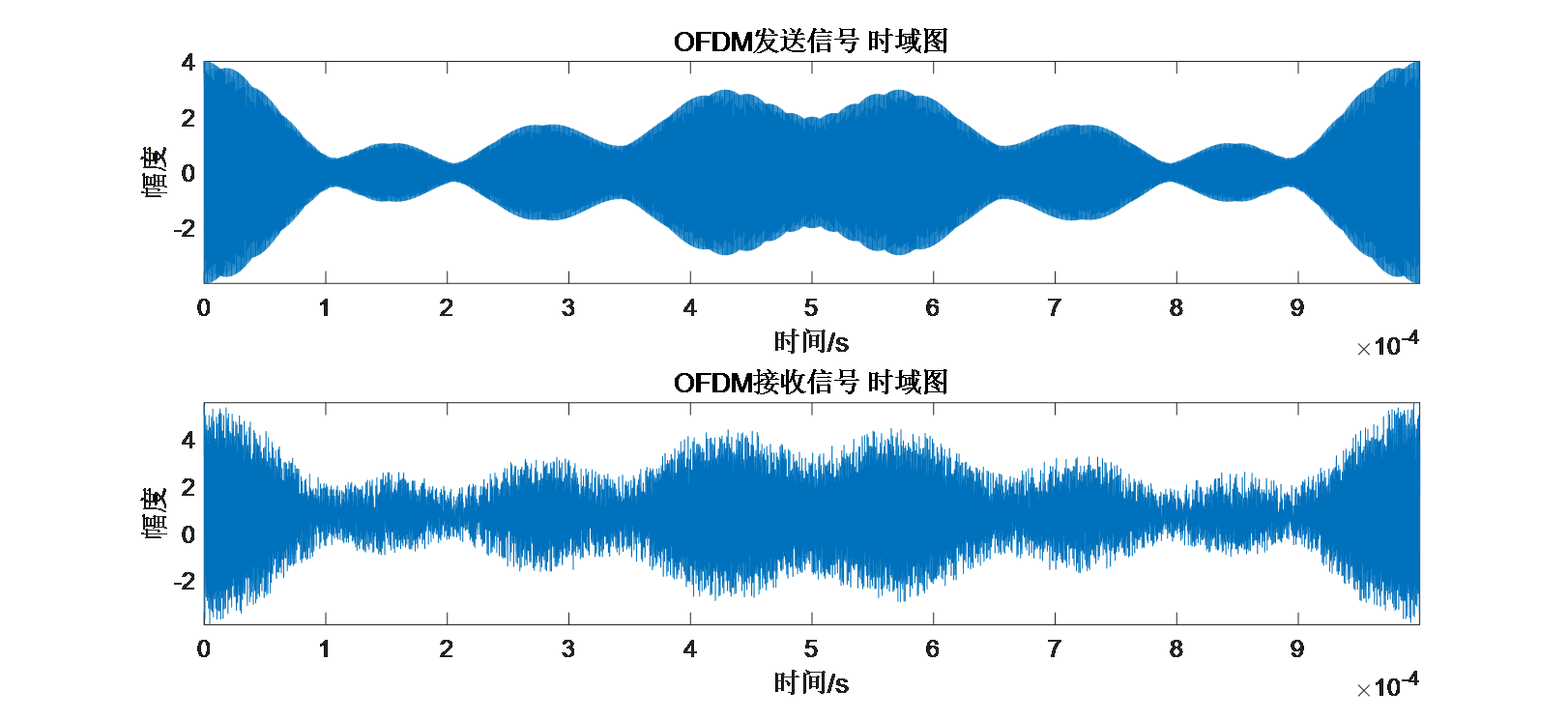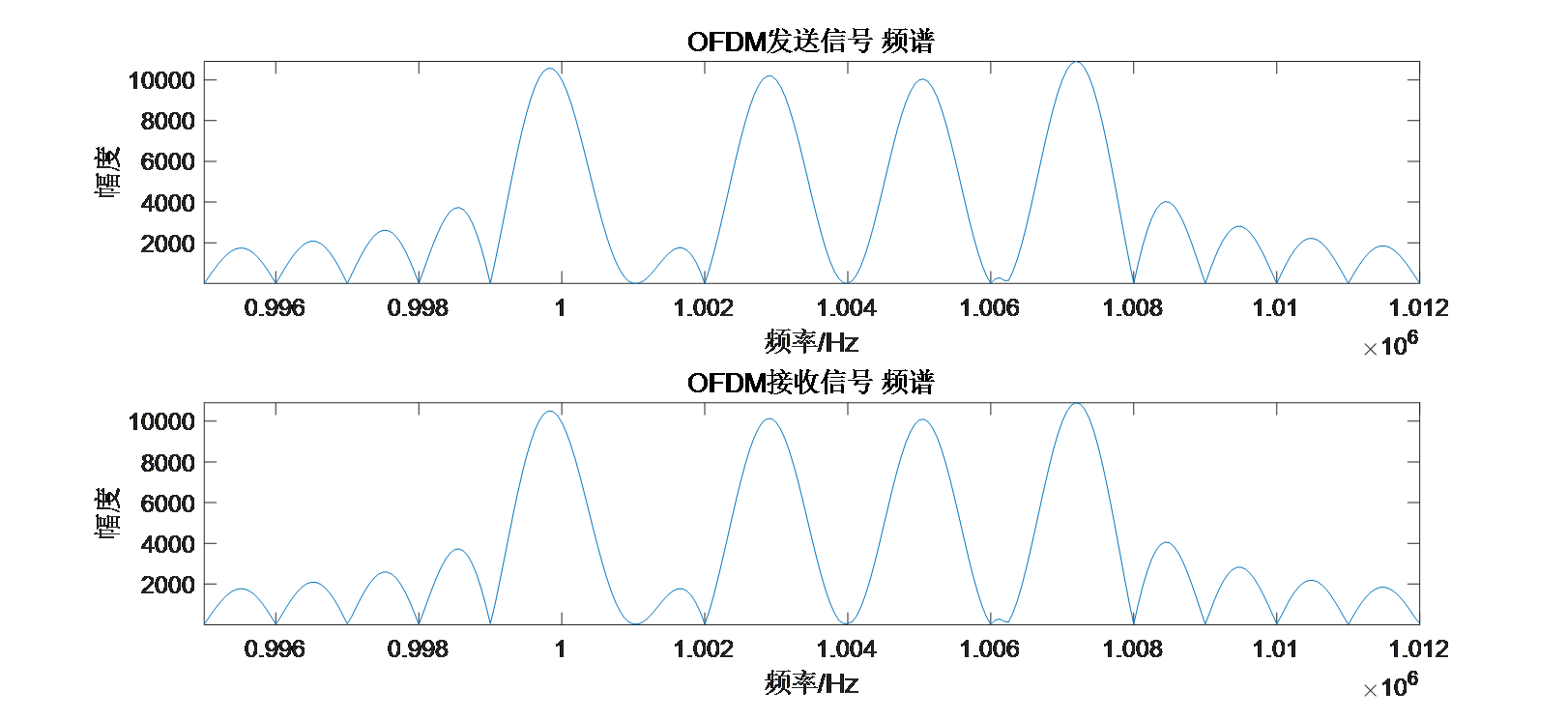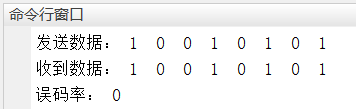展开全文通信原理 信号处理 matlab

千次阅读 2021-05-10 23:03:45

0 概述

基带传输信道中的信号是未经过调制的，载波传输信道中的信号是经过调制的。

数字调制是指数字基带信号变化的过程，通过待传输的数字基带信号去控制载波的参量，使之随数字基带信号的变化而变化的过程。数字调制的目的是使基带信号适合于在信道上传输
数字解调是指把已调信号在接收端变回数字基带信号。

数字调制系统模型如下图所示，与基带传输系统相比增加了调制和解调部分，其余部分基本相同。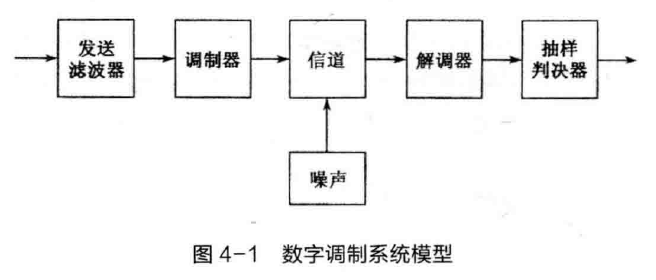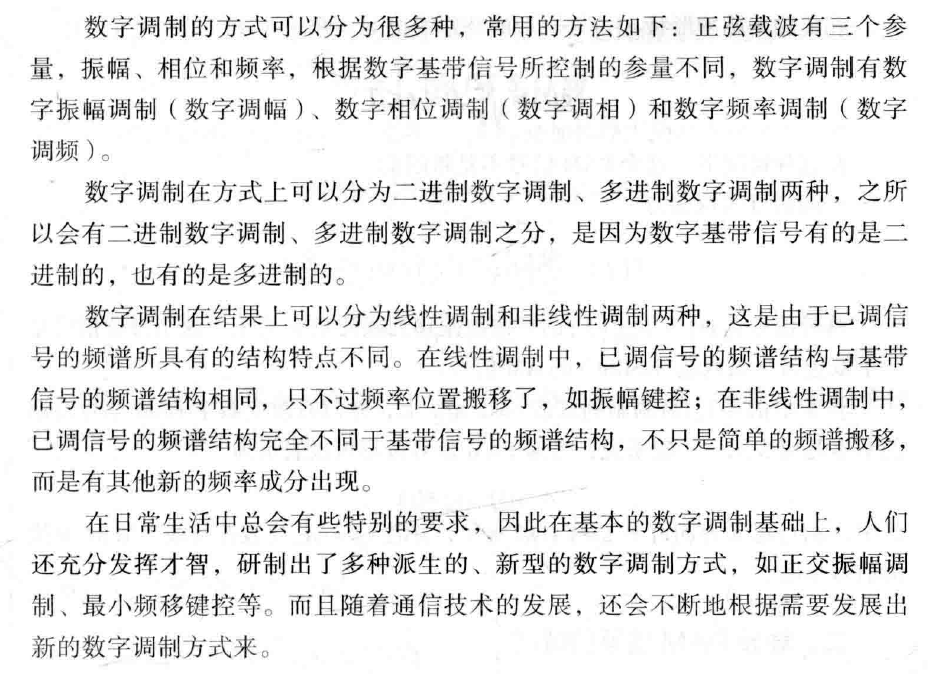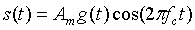其中fc为载波频率，Am是第m个波形的幅度，g(t)是决定传输信号的谱特性的某种脉冲。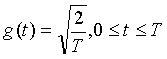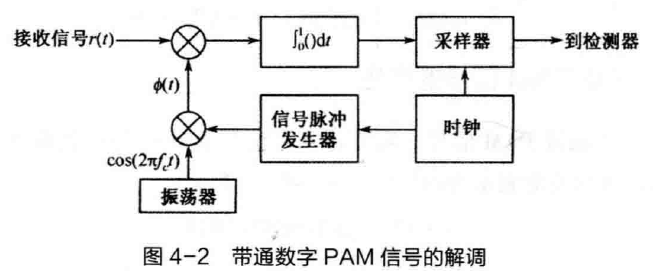接收信号为：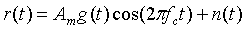其中n(t)是带通噪声过程。

上图中：φ(t)=g(t)cos(2πfct)

接收信号与φ(t)做互相关，得到的输出为：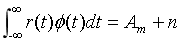得到Am+n后，使用与基带PAM信号解调类似的方式（根据欧氏距离判定），检测器即可输出当前认为接收到的信号值。

MATLAB程序

clc;
clear;
close all;

symbol = 100000;% 每种信噪比下的发送符号数
T = 1;			% 符号周期/s
fs = 100;		% 采样频率/Hz
ts = 1/fs;		% 采样时间间隔
t = 0:ts:T-ts;	% 时间矢量
fc = 10;		% 载波频率/Hz
c = sqrt(2/T)*cos(2*pi*fc*t);% 载波信号

% 绘制载波波形
figure;
subplot(4,1,1);plot(t,-3*c);legend('Amp = -3');axis([-inf inf -5 5]);
subplot(4,1,2);plot(t,-1*c);legend('Amp = -1');axis([-inf inf -5 5]);
subplot(4,1,3);plot(t, 1*c);legend('Amp =  1');axis([-inf inf -5 5]);
subplot(4,1,4);plot(t, 3*c);legend('Amp =  3');axis([-inf inf -5 5]);

graycode = [0 1 3 2];           % Gray编码规则
EsN0 = 0:12;                    % 信噪比，Es/N0
snr1 = 10.^(EsN0/10);			% 信噪比转换为线性值
msg  = randi([0 3],1,symbol);   % 消息比特（0 1 2 3 组成的随机序列）
msg1 = graycode(msg+1);         % Gray映射：0 1 2 3 -> 0 1 3 2

msg2 = zeros(1,length(msg1));
for k = 1:length(msg1)
if(msg1(k) == 0)
msg2(k) = -3;
elseif(msg1(k) == 1)
msg2(k) = -1;
elseif(msg1(k) == 2)
msg2(k) = 1;
elseif(msg1(k) == 3)
msg2(k) = 3;
end
end

% 载波调制
tx = zeros(1,length(msg2)*length(t));
for k = 1:length(msg2)
for p = 1:length(c)
tx((k-1)*length(t)+p) = msg2(k)*c(p);% 幅值*载波信号
end
end

slow = norm(tx).^2/symbol;	% 求每个符号的平均功率

bit_error_percent = zeros(1,length(EsN0));
for i = 1:length(EsN0)
sigma = sqrt(slow/(2*snr1(i)));				% 根据符号功率求噪声功率
rx = tx + sigma*rand(1,length(tx));    		% 加入AWGN
rx1 = reshape(rx,length(c),length(msg2));	% 把数据变为一个符号是一列的格式
r00 = (c * rx1)/length(c);          		% 相关器输出，每次求length(c)个连续采样点与rx的互相关
% 判决：欧氏距离最接近
msg_demodulation = zeros(1,symbol);
for k = 1:symbol
D0 = abs(r00(k) - (-3));% 计算欧氏距离
D1 = abs(r00(k) - (-1));
D2 = abs(r00(k) - ( 1));
D3 = abs(r00(k) - ( 3));
Dis(1,1:4) = [D0 D1 D2 D3];
[x,y] = min(Dis);% 求最小值的位置
msg_demodulation(k) = y-1;
end
decmsg = graycode(msg_demodulation+1);% Gray逆映射
bit_error_cnt = 0;
for k = 1:symbol
if(decmsg(k) ~= msg(k))
% 当判定的接收比特与发送比特不一致时，认为判定错误
bit_error_cnt = bit_error_cnt + 1;
end
end
bit_error_percent(i) = bit_error_cnt/symbol;
end
figure;
plot(EsN0,bit_error_percent,'-o');
xlabel('Es/N0');
ylabel('误比特率Pe');
legend('仿真结果');

结果：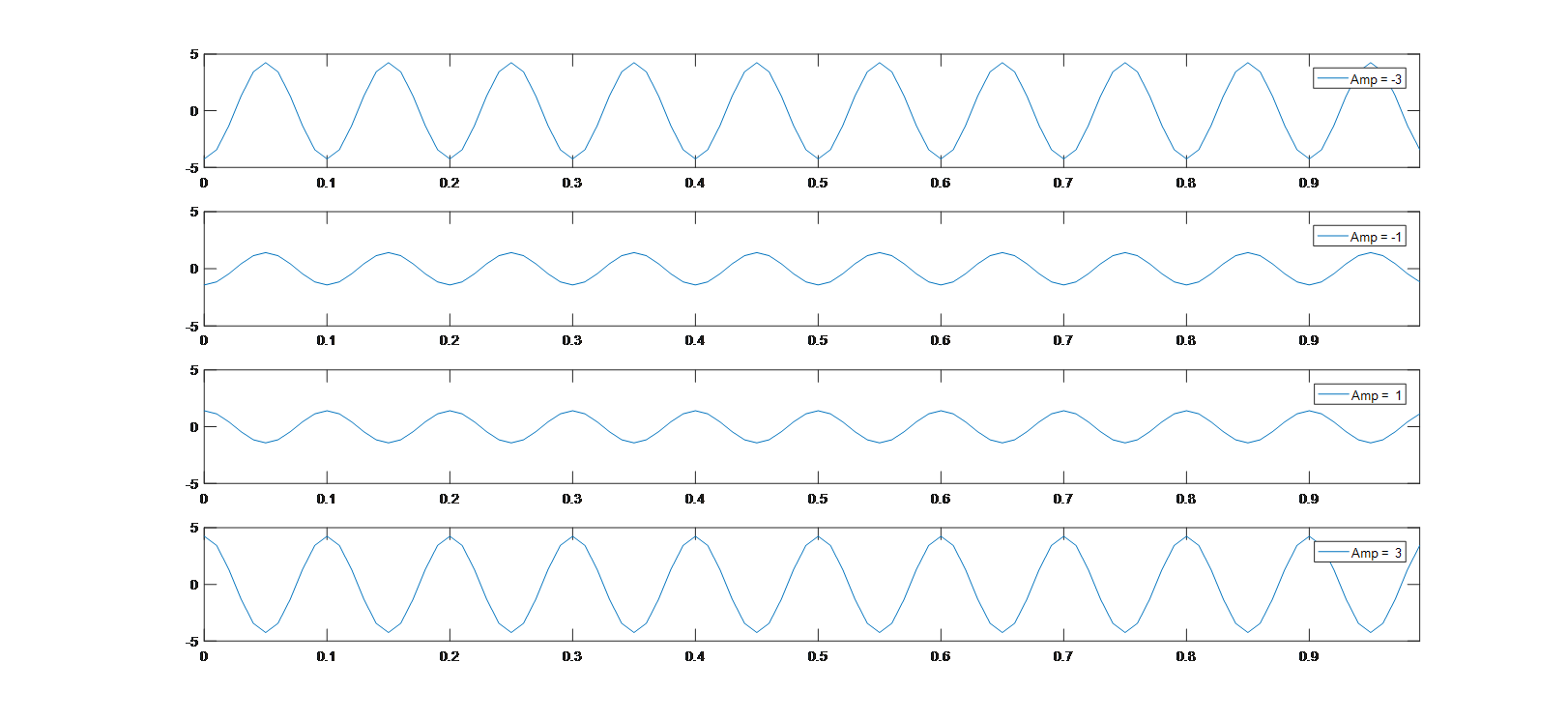误比特率结果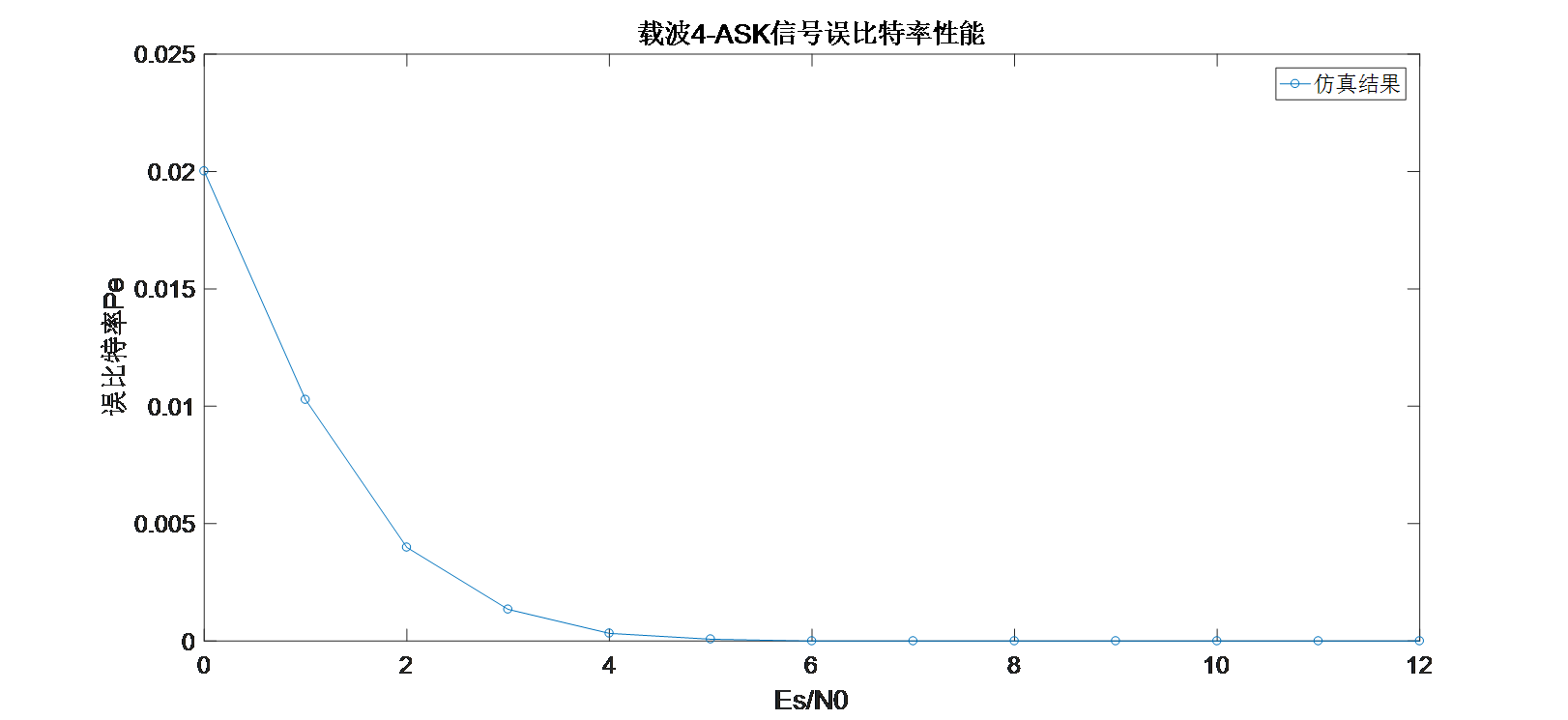展开全文通信原理 数字通信 信号处理
• 数字信号载波传输PSK1 PSK调制2 PSK解调3 载波PSK信号的仿真 1 PSK调制 2 PSK解调 3 载波PSK信号的仿真 MATLAB程序 仿真载波8-PSK信号 clc; clear; close all; symbol = 10; % 发送符号数 T = 1; % 符号周期...

1 PSK调制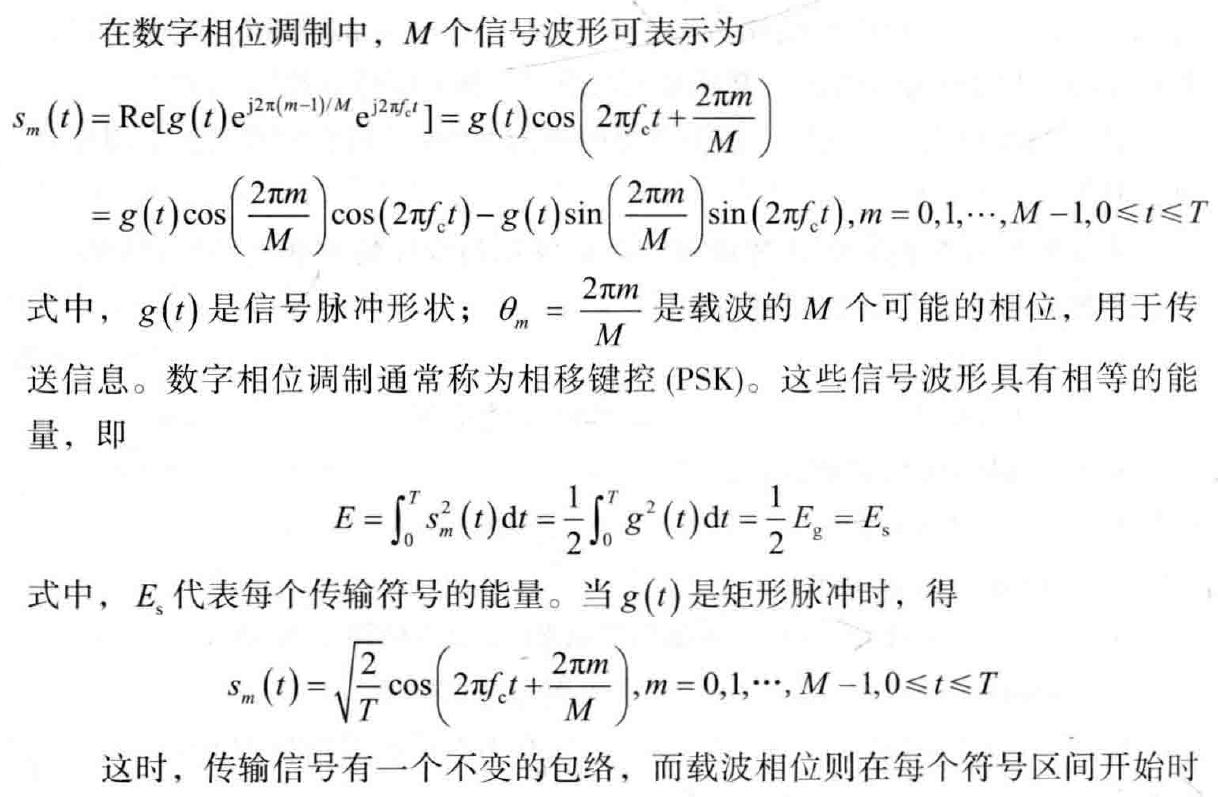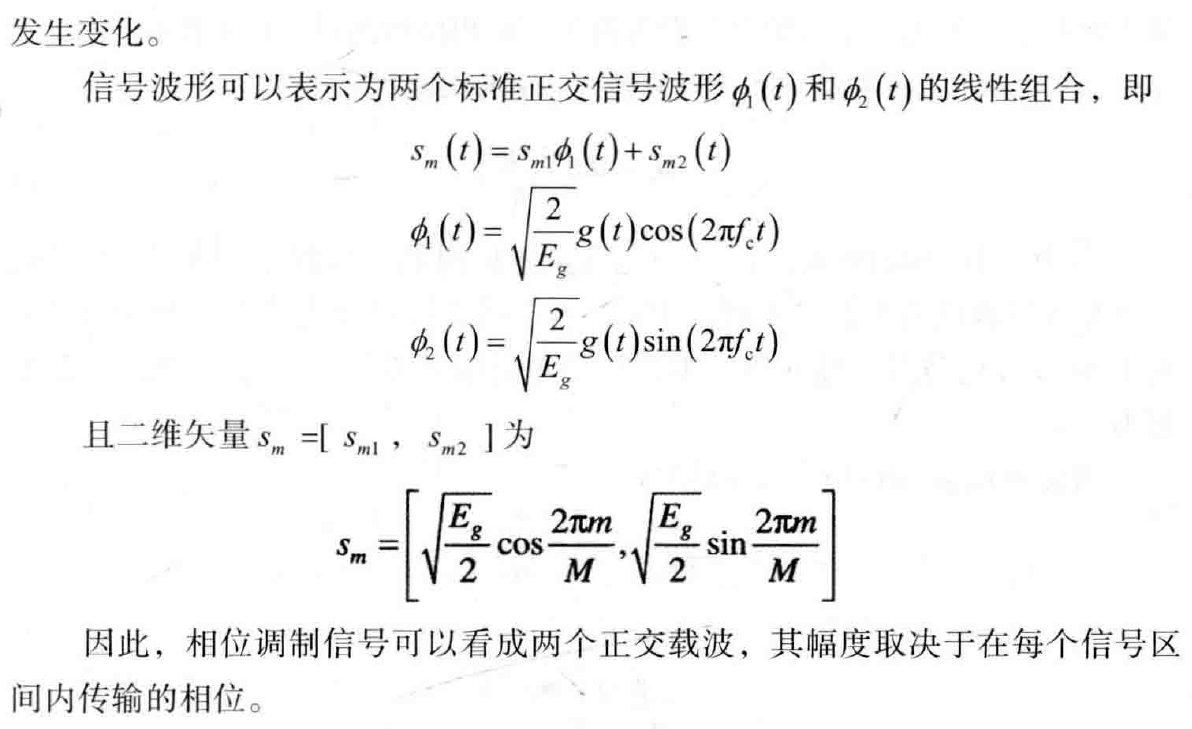2 PSK解调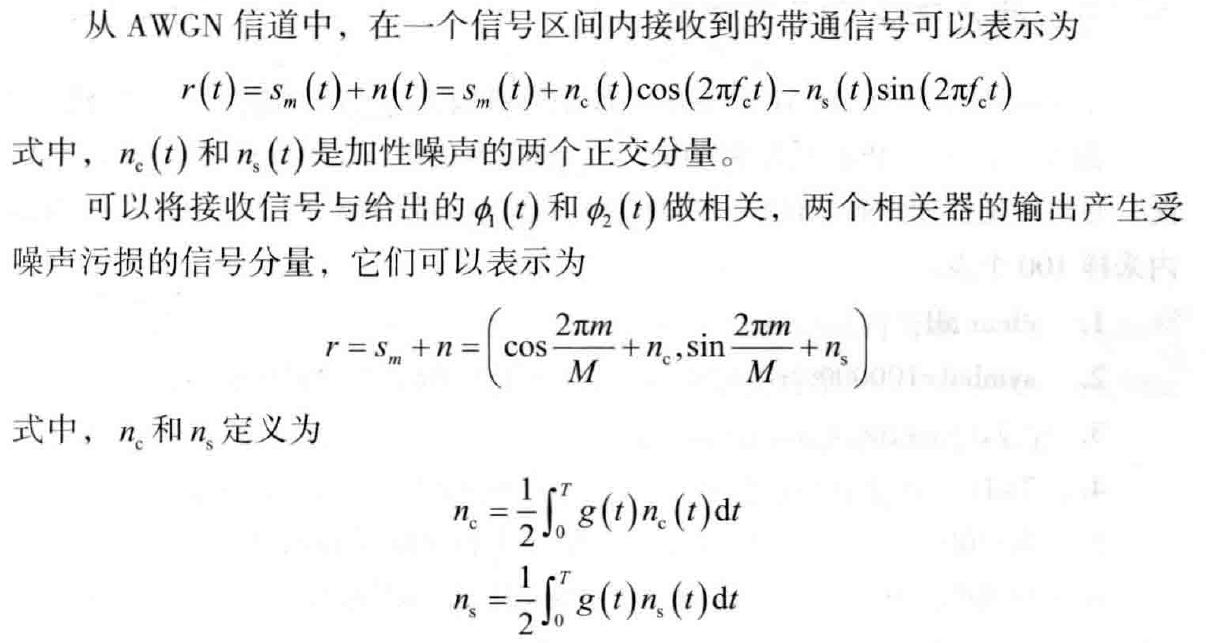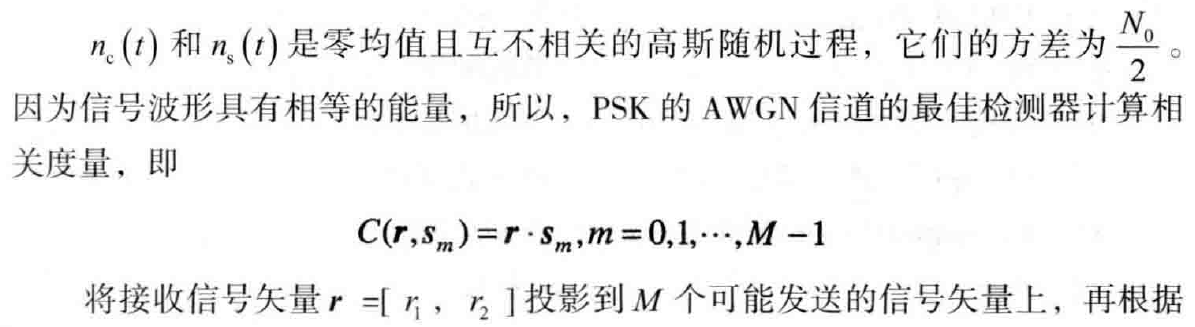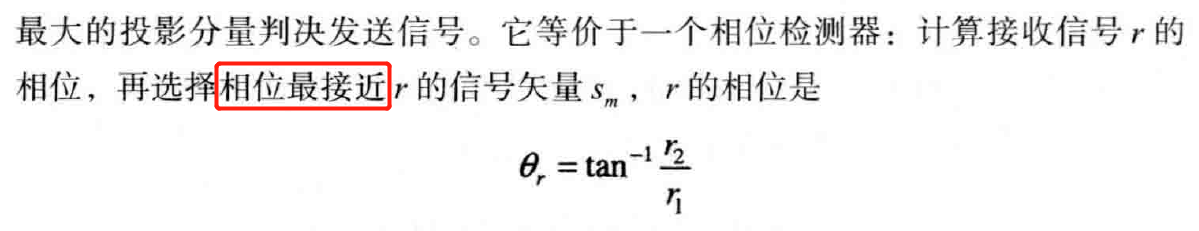3 载波PSK信号的仿真

MATLAB程序

仿真载波8-PSK信号

clc;
clear;
close all;

symbol = 10;	% 发送符号数
T  = 1;			% 符号周期/s
fs = 100;		% 采样频率/Hz
ts = 1/fs;		% 采样时间间隔
t  = 0:ts:T-ts;	% 时间矢量
fc = 10;		% 载波频率/Hz
c  = sqrt(2/T)*exp(1j*2*pi*fc*t);	% 载波信号
c1 = sqrt(2/T)*cos(2*pi*fc*t);		% 同相载波
c2 = -sqrt(2/T)*sin(2*pi*fc*t);		% 正交载波
M  = 8;								% 8-PSK

% 绘制载波波形
theta = 2*pi/M * (0:M-1);	% M种 载波相位
c_signal = zeros(M,length(t));
for k = 1:M
c_signal(k,:) = real( c .* exp(1j*theta(k)) );
end
figure;
subplot(8,1,1);plot(t,c_signal(1,:));legend('theta = 2*pi* 0/8');axis([-inf inf -5 5]);
subplot(8,1,2);plot(t,c_signal(2,:));legend('theta = 2*pi* 1/8');axis([-inf inf -5 5]);
subplot(8,1,3);plot(t,c_signal(3,:));legend('theta = 2*pi* 2/8');axis([-inf inf -5 5]);
subplot(8,1,4);plot(t,c_signal(4,:));legend('theta = 2*pi* 3/8');axis([-inf inf -5 5]);
subplot(8,1,5);plot(t,c_signal(5,:));legend('theta = 2*pi* 4/8');axis([-inf inf -5 5]);
subplot(8,1,6);plot(t,c_signal(6,:));legend('theta = 2*pi* 5/8');axis([-inf inf -5 5]);
subplot(8,1,7);plot(t,c_signal(7,:));legend('theta = 2*pi* 6/8');axis([-inf inf -5 5]);
subplot(8,1,8);plot(t,c_signal(8,:));legend('theta = 2*pi* 7/8');axis([-inf inf -5 5]);

EsN0 = 20;						% 信噪比，Es/N0
snr1 = 10.^(EsN0/10);			% 信噪比转换为线性值
% msg  = randi([0 7],1,symbol);	% 消息比特（随机）
msg  = [0 1 4 6 6 2 0 3 5 7];	% 消息比特

% 8-PSK载波调制
tx = zeros(1,length(msg)*length(t));
for k = 1:length(msg)
for p = 1:length(c)
tx((k-1)*length(t)+p) = c_signal(msg(k)+1,p);% 幅值+载波信号
end
end
figure;
subplot(2,1,1);plot(tx);axis([-inf inf -5 5]);title('8-PSK 发送信号  0 1 4 6 6 2 0 3 5 7');
disp(['发送数据： ' num2str(msg)]);

% 信道传输
slow  = norm(tx).^2/symbol;					% 求每个符号的平均功率
sigma = sqrt(slow/(2*EsN0));				% 根据符号功率求噪声功率
rx    = tx + sigma*rand(1,length(tx));		% 加入AWGN
subplot(2,1,2);plot(rx);axis([-inf inf -5 5]);title('8-PSK 接收信号');

rx1 = reshape(rx,length(t),length(msg));	% 把数据变为一个符号是一列的格式
r1  = (c1 * rx1)/length(t);          		% 相关器输出，分别求与正交信号的互相关
r2  = (c2 * rx1)/length(t);
r   = r1 + 1j*r2;
% 判决：欧氏距离最接近
msg_demodulation = zeros(1,symbol);
Angle = zeros(1,symbol);
for k = 1:symbol
Dis = zeros(1,M);
for n = 1:M
% 求复数相角，结果在[-pi,pi]，转换为[0,2*pi]
if(angle(r(k)) < 0)
Angle(k) = 2*pi + angle(r(k));
else
Angle(k) = angle(r(k));
end
% 计算欧氏距离
Dis(n) = abs(Angle(k) - (2*pi * 1/8 *(n-1)));
end
%     disp(['atan: ' num2str(Angle(k))]);
%     disp(['Dis : ' num2str(Dis)]);
[x,y] = min(Dis);% 求最小值的位置
msg_demodulation(k) = y-1;
end
disp(['收到数据： ' num2str(msg_demodulation)]);

bit_error_cnt = 0;
for k = 1:symbol
if(msg_demodulation(k) ~= msg(k))
% 当判定的接收比特与发送比特不一致时，认为判定错误
bit_error_cnt = bit_error_cnt + 1;
end
end
bit_error_percent = bit_error_cnt/symbol;
disp(['误码率： ' num2str(bit_error_percent)]);

结果：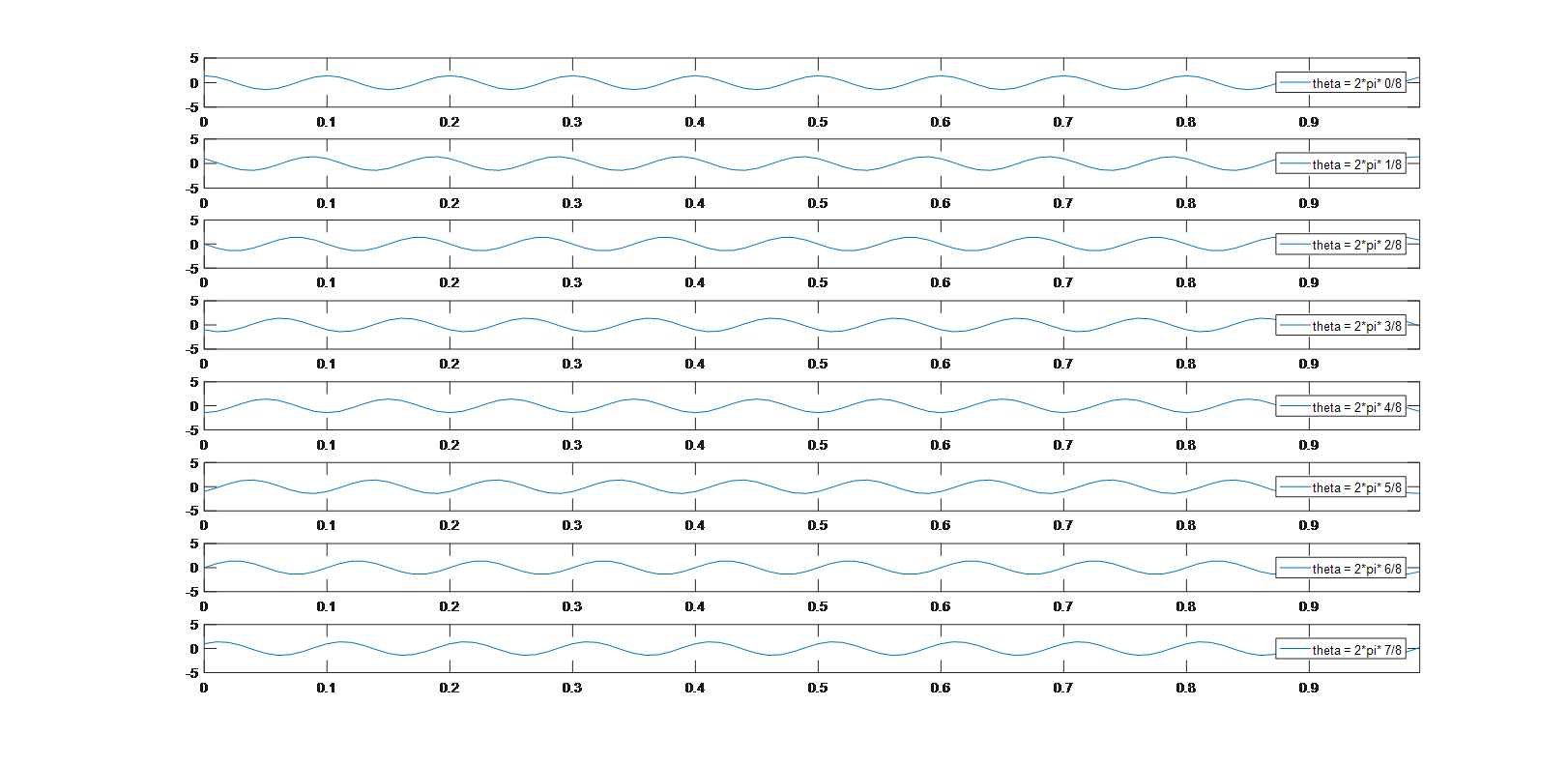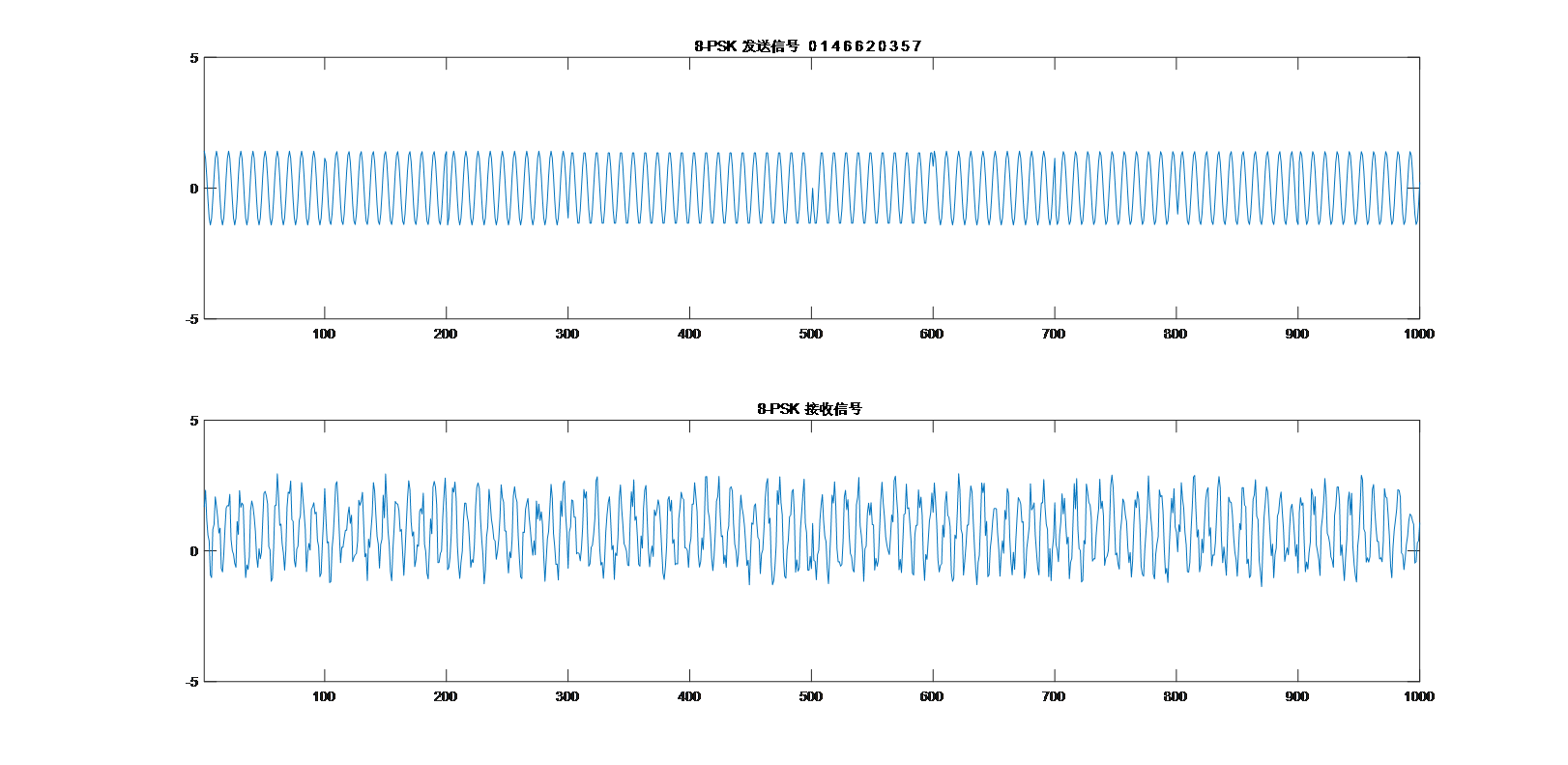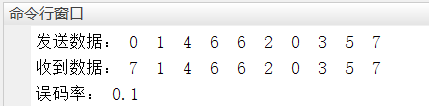展开全文通信原理 信号处理 matlab
• 数字信号载波传输QAM1 QAM调制2 QAM解调3 QAM星座图 1 QAM调制 2 QAM解调 3 QAM星座图 星座图有两根轴。水平X轴与同相载波相关，垂直Y轴与正交载波相关。图中每个点，可以包含4条信息：点在X轴的投影定义了...

1 QAM调制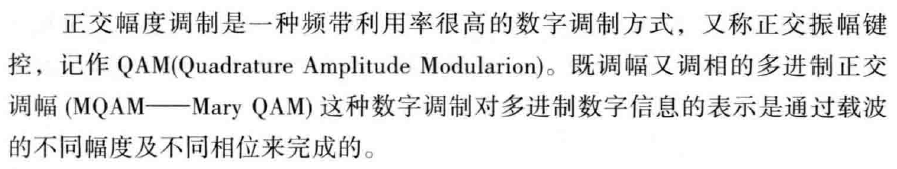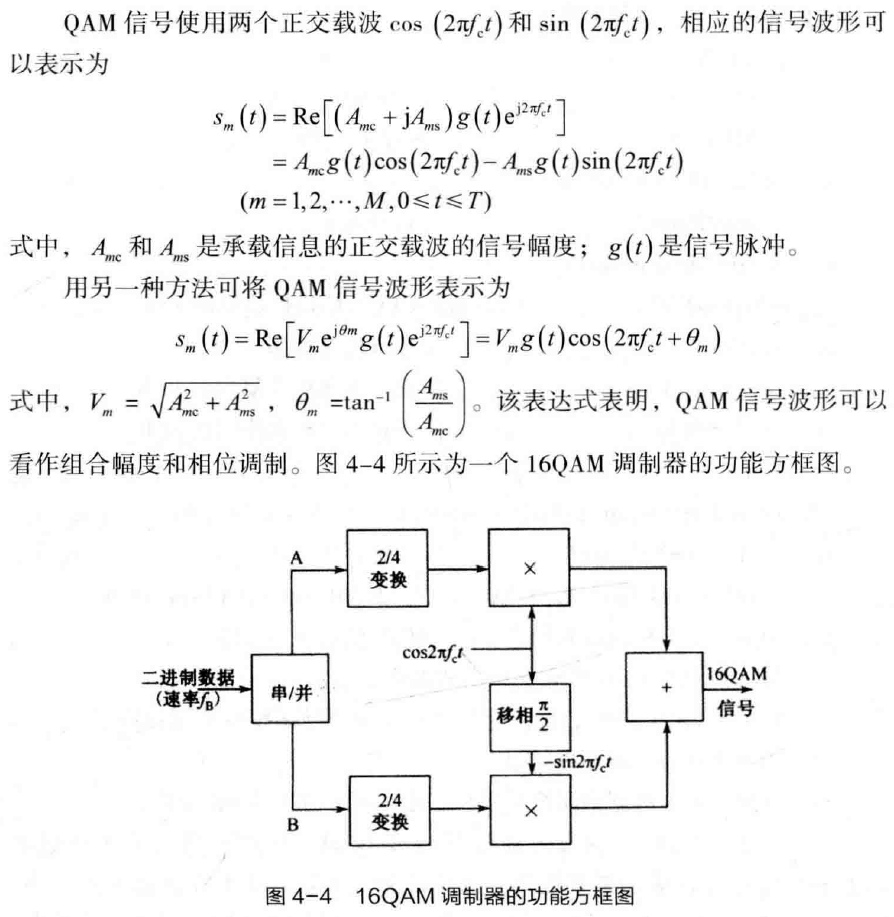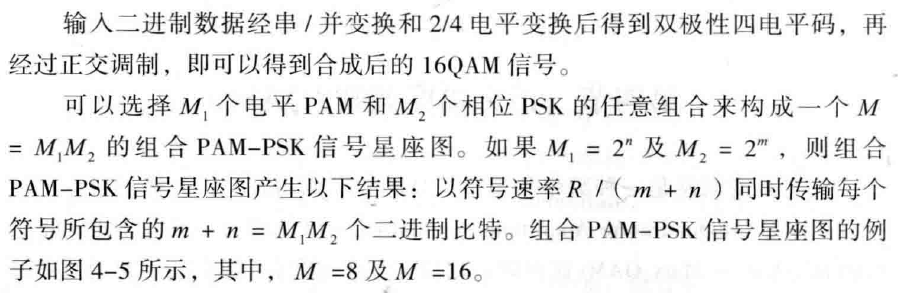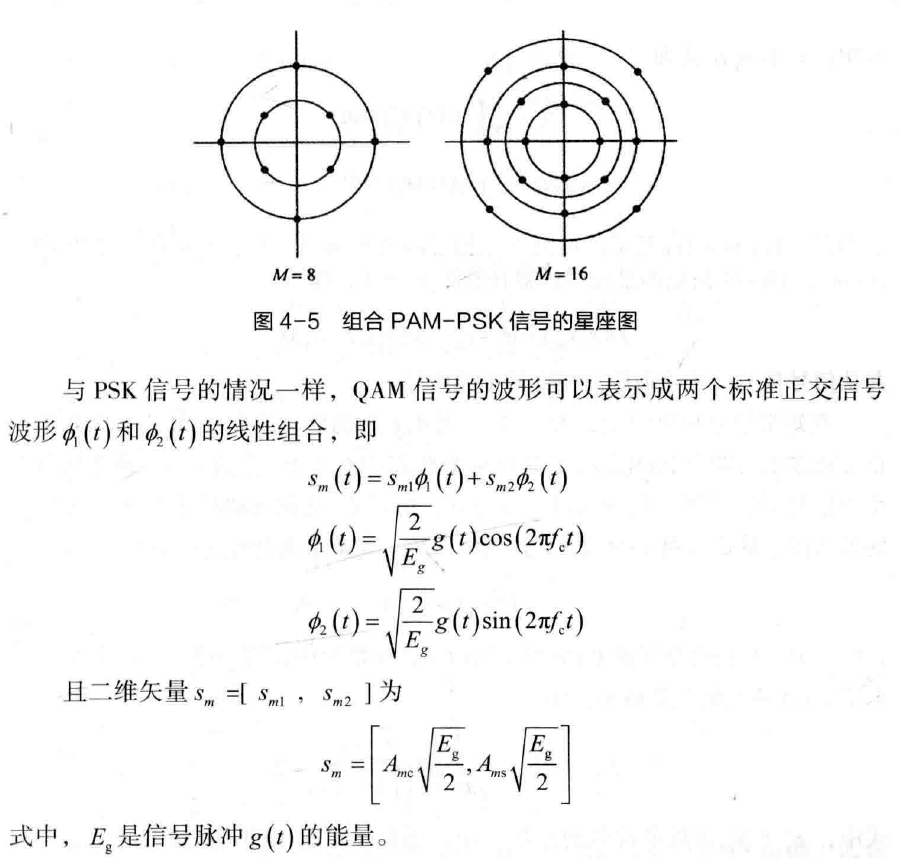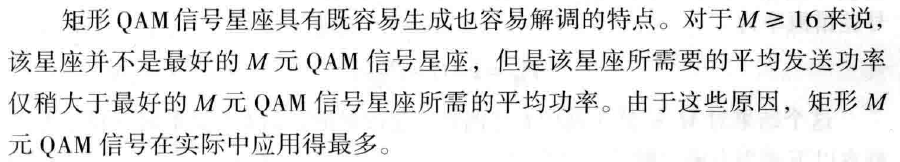2 QAM解调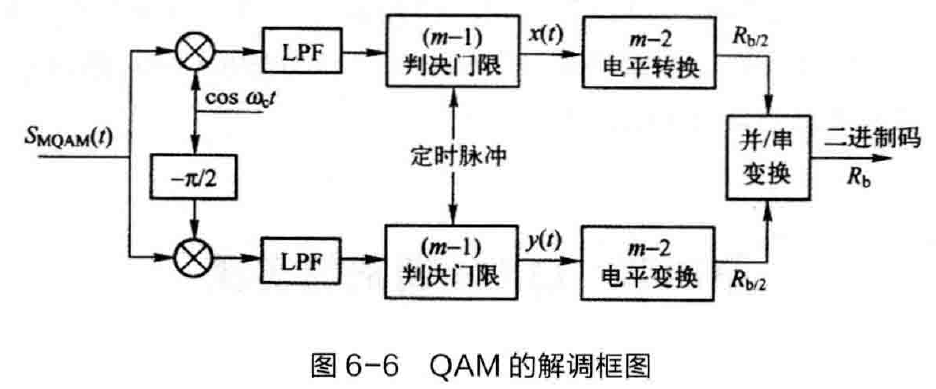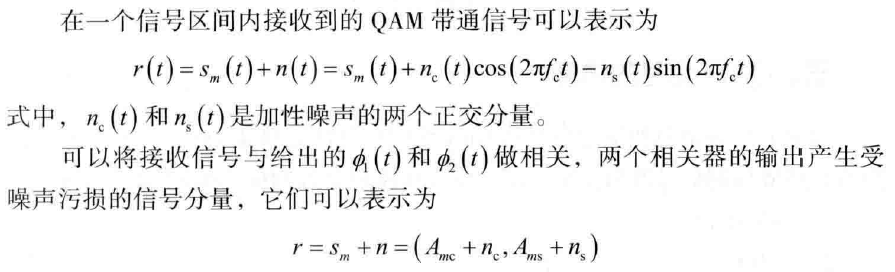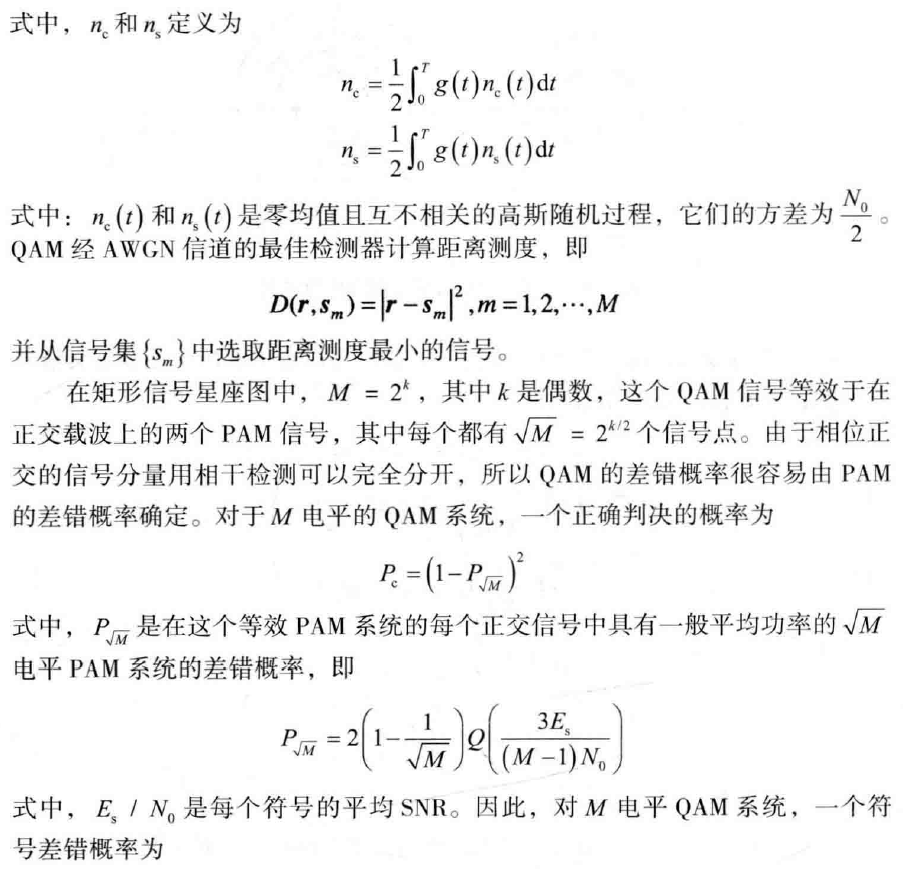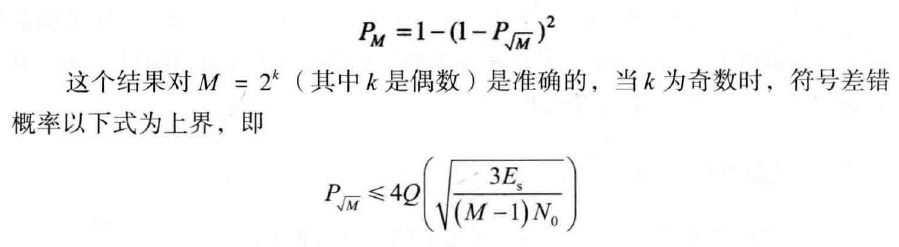3 QAM星座图

星座图有两根轴。水平X轴与同相载波相关，垂直Y轴与正交载波相关。图中每个点，可以包含4条信息：点在X轴的投影定义了同相成分的峰值振幅，点在Y轴的投影定义了正交成分的峰值振幅，点到原点的连线(向量)长度是该信号元素的峰值振幅，连线和X轴之间的角度是信号元素的相位

例如64QAM，符号有64个，等于2的6次方，因此每个符号需要6bit的二进制来表示。根据幅度和相位的不同，每个信号元素所在的位置就不同，从其中一个点跳到另一个点，就意味着相位调制和幅度调制同时完成了。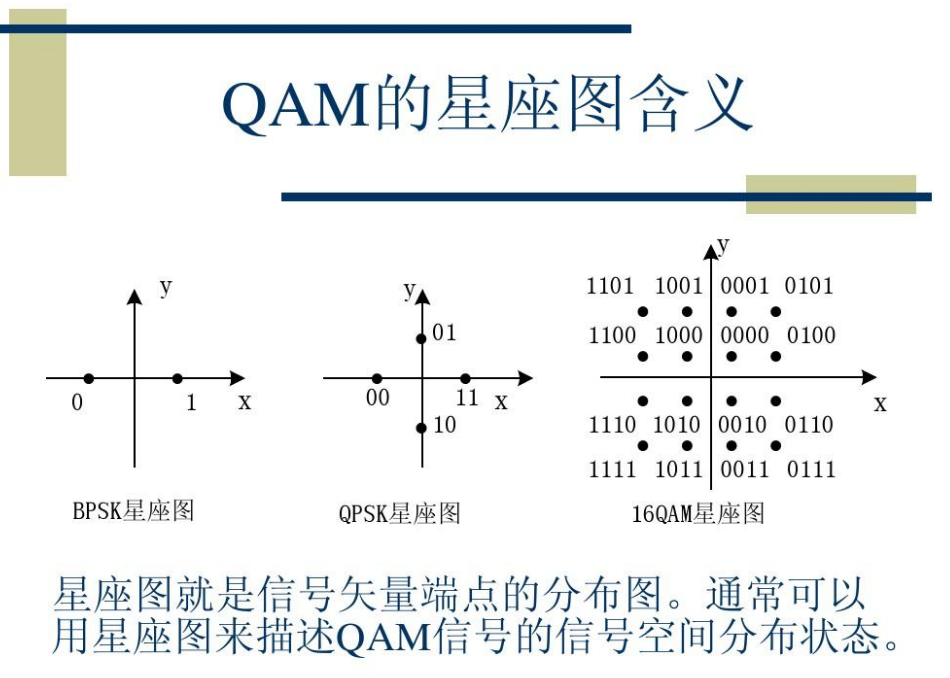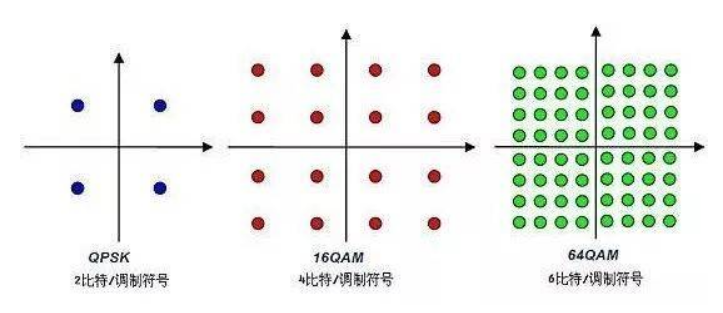展开全文通信原理 调制解调
• 数字信号载波传输FSK1 FSK调制2 FSK解调3 载波FSK信号的仿真 1 FSK调制 2 FSK解调 3 载波FSK信号的仿真 MATLAB程序 仿真载波4-FSK信号 clc; clear; close all; symbol = 10; % 发送符号数 M = 4; % 4-...通信原理 信号处理 matlab
• 因此，采用载波并行传输信号能够有效对抗单一载波易受干扰的问题。而且，将高速的串行码流分散到个并行的低速码流中传输，有利于使用高阶调制方式。但个码流如何“并行不悖”的传输是个问题。 OFDM就是采用...算法
• 台电脑共享一个传输媒介，这种方法叫“载波侦听路访问”，简称CSMA，全称Carrier Sense Multiple Access 。 Carrier （载体）指的是运输数据的共享媒介，以太网的“载体”是铜线（。。。），WiFi的“载体”是...CSMA 载波监听多路访问
• 一种lte上行链路分步式动态子载波分配方法【技术领域】 本发明涉及移动通信技术资源分配领域，尤其是一种LTE上行链路动态子载波分 配方法。【背景技术】 目前LTE作为最主要的4G技术，对于其频谱资源分配...
• T1载波是把24个话音信道路复用在一条高速信道上，每个信道包含7位的数据和1位的控制信令位，此外加入一位帧同步位组成基本帧。 其中，用户的开销为24×1(控制位)+1(基本帧)=25 b 总开销为：(7+1)×24+1=193 b 因此...e1载波的数据速率是
• 二 单载波频域均衡技术 2.1 单载波频域均衡系统简介 在对抗径衰落信道方面,...二单载波频域均衡技术 2.1 单载波频域均衡系统简介 在对抗径衰落信道方面,基...在分析了正交频分复用(OFDM)技术优缺点的基础上提出...
• 载波频率

2021-01-13 11:15:33
载波频率是在信号传输的过程中，并不是将信号直接进行传输，而是将信号负载到一个固定频率的波上，这个过程为加载，这样的一个固定频率。严格的讲，就是把一个较低的信号频率调制到一个相对较高的频率上去，这被低频...
• 1. 为什么需要载波聚合？一般来说，要提升网速或者容量，有下面几个思路：建更的基站：这样一来同一个基站下抢资源的人就少了，网速自然就上去了。但缺点是投入太大了，运营商肯定不会做亏本的买卖。提升频谱效率...
• PLC（Power Line Carrier）电力线载波简介 简介 电力载波通讯PLC,是指现有电力线上进行模拟信号和数据载波通讯Power Line Communication.随着技术的进步,人们在这一领域进行了长时间的大量的研究和试验.近几年来PLC...stm32 物联网 网络协议 需求分析
• 科普：谈谈载波聚合

千次阅读 2020-12-20 12:06:11
话说随着智能手机的普及和移动互联网的发展，各种...那就是“载波聚合”。载波聚合到底是怎样实现速率飙升的呢？双连接技术又是怎样在载波聚合的基础上锦上添花的？下文即将揭晓。1为什么需要载波聚合？一般来说，要...
•网络 网络协议
• 1为什么需要载波聚合？一般来说，要提升网速或者容量，有下面几个思路：建更的基站：这样一来同一个基站下抢资源的人就少了，网速自然就上去了。但缺点是投入太大了，运营商肯定不会做亏本的买卖。提升频谱效率：...
• 关于子载波间隔的总结

万次阅读 2021-03-29 11:03:10
OFDM系统在时域上的资源占用取决于基频子载波间隔 本质上从傅里叶变换和FFT变换来解释的。 对于傅里叶变换来说，时域积分的时间长度对应频率分辨率。也就是说T为F的倒数。 对于DFT来说，是将时域T分割成N块，为了...
• 子载波间隔

千次阅读 2021-06-23 09:44:37
一个子信道有一个或者个子载波，子载波就是一个个载波，比如100MHz带宽里，假设15KHz是一个子载波，这里边有很个子载波。 (这里子载波数并不是100M/15K。例如，小区带宽配置为20MHz，对应的RB[资源块]为100个，...网络通信
• 通过载波频率来区分子信道是一种常用的路复用的技术，即频分路复用FDM技术。 比如，假设单路语音信号只需要20K的基带信号带宽，移动通信系统提供20M的带宽，这样20M的频谱带宽就可以同时传输20M/10K=2000路信号...
• 刘畅安1，胡芳仁1, 2，刘昕1(1. 南京邮电大学 光电工程学院，江苏 南京 ...对载波相位恢复算法进行了研究，在此基础上提出了利用小波变换中的小波分解和小波重构的方法来减少相位噪声，通过结果比对选取最佳分解次数...
• 5.2.1 载波频偏的捕获 A．前导序列估计由于发送端和接收端载波频率的不同，每一个采样信号在时间t时包含一个未知的相位因素ej2??fct，这里?fc指的是未知的载波频偏。这个未知的相位因素在接收端必须被估计和补偿，...
• 通过不同的调制方式（诸如FSK、QAM等等），可以在一个码元符号上负载个bit位信息。举个例子，下图是4QAM（即QPSK）调制的全部四种码元符号，一种符号可以带两个bit的信息。 3. 符号率 符号率也就是码元速率，...
• 网线上有计算机发送数据时，网线的传输资源就被占用了。但是这个跟计算机内部总线被占用不同，总线的占用有cpu做调节，所以不会争抢，但是网线是没有cpu来调节的，所以连接在网线上的各个主机必须要遵循一个协议，来...
• 以太网—CSMA/CD 载波侦听路访问/碰撞检测一、参考网址二、工作原理三、二进制指数退避算法 小白刚开始学习以太网的知识，综合了几篇文章，记录了一些知识点和自己的理解，不知道有没有错误的地方，多多指教~ 一、...
• 收到的比特流 中提取 位同步信号 使用曼彻斯特编码可以方便提取位同步信号 曼彻斯特编码的 缺点 ：所占的频带宽度比原始的基带信号加倍了（每秒的传输码元数加倍了） CSMA / CD 协议要点 载波监听 多点接入 碰撞检测...
• 类似于载波聚合(CA) ，其目标是利用载波之间的无线资源来提高终端吞吐量。DC与CA之间区别主要在于它们应用场景和实现的不同。整理：kangguoying20200822根据3GPP 36.300定义双连接(DC)只是一种操作模式;在这种...
• 在讨论详细的NR下行链路和上行链路传输方案之前，将在本章中对NR的基本时频传输资源进行描述，包括带宽部分，补充上行链路，载波聚合，双工方案，天线端口和 准代管。 7.1　传输机制 由于OFDM对时间分散的鲁棒性以及......